Next: Summary Results from the Up: Supporting Theorems Previous: An Example of Cyclical

## WaterSluice Software Engineering Methodology

In this section, a family of theorems are presented that pertain to the WaterSluice software engineering methodology. The WaterSluice software engineering methodology will find solutions in static environments and dynamic environments including dynamic environments that have the non-monotonic property. If a solution exists and the WaterSluice software engineering methodology finds the solution, the best case performance is O(1), the worst case performance is O(N), while the average case performance is an order-of-magnitude less than N where N is the total number of steps in the environment.

Theorem 4 (WaterSluice: Static Complete)   The WaterSluice software engineering methodology is static complete.

Let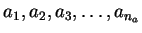be the na steps leading to the possible analysis A with a1 being the initial problem statement. Let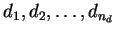be the nd steps leading to the possible design D. Let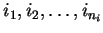be the ni steps leading to the possible implementation I. Let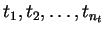be the nt steps leading to the possible testing T with tn<<1931>>4 being the final system acceptance test. Define an environment that consists of the four planes of analysis, design, implementation, and testing with each lower plane being a refinement of the higher planes. In this environment priorities are represented as different in the size of a step. A larger circle represents a higher priority than a smaller circle. Define the solution as the tree, or in a more general sense, the directed acyclic graph, of all steps from all four planes used in the final system. Define a priority queue of possible next steps PQ. Initially the priority queue PQ contains the initial step a1 represented by the sequence S1. Define a function, next, over all possible steps, which generates all possible next steps given the current solution sequence, evaluates the total value, and pushes the ordered result onto the priority queue PQ. Only high priority steps are keep in the queue PQ. See Figure 5.13 on pagefor a visual representation of the space. Here a larger circle represents a higher priority step. See figures 5.13, 5.15, 5.16, 5.17, and 5.18 on pagesthroughfor a visual guide of the reasoning. The numbers associated with the steps represent the order visited by the methodology.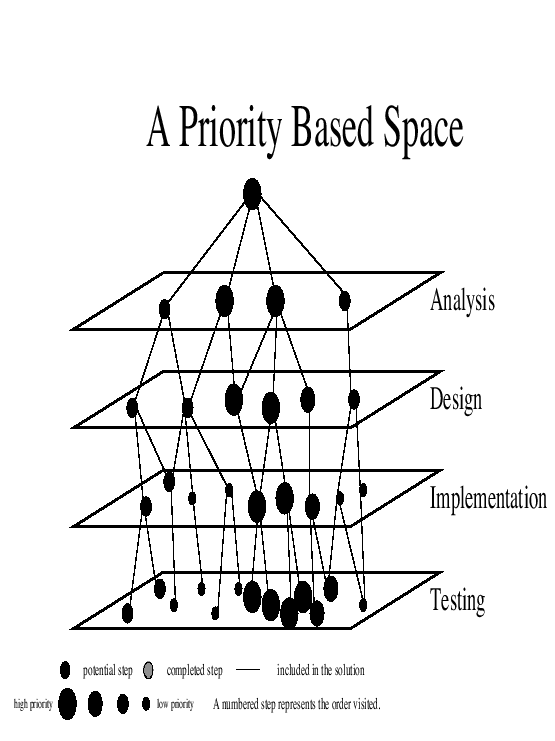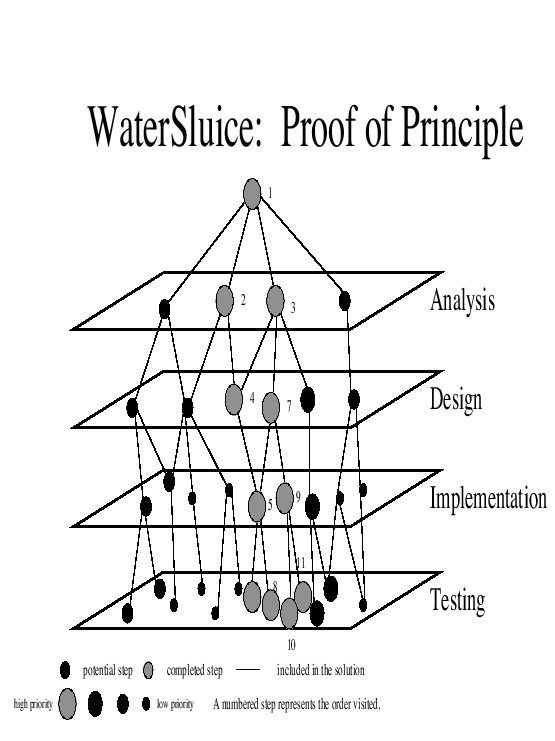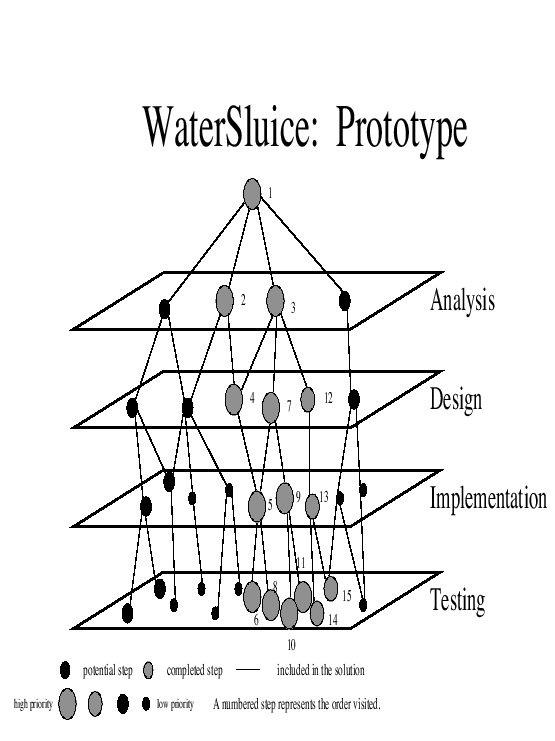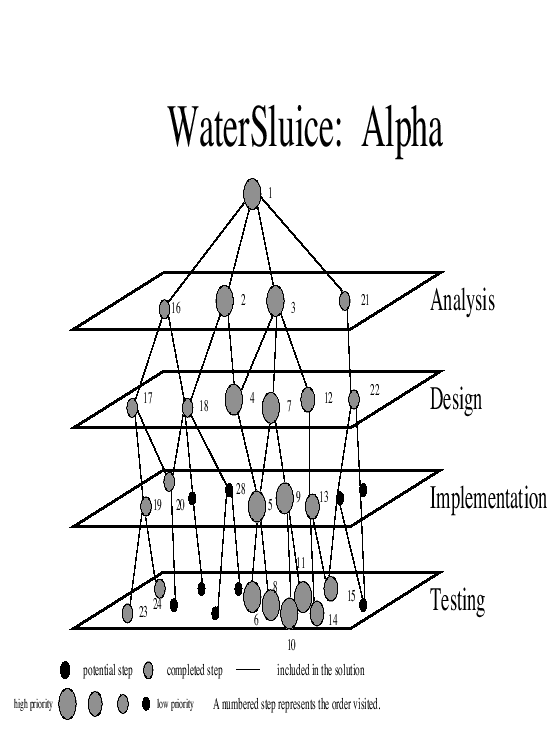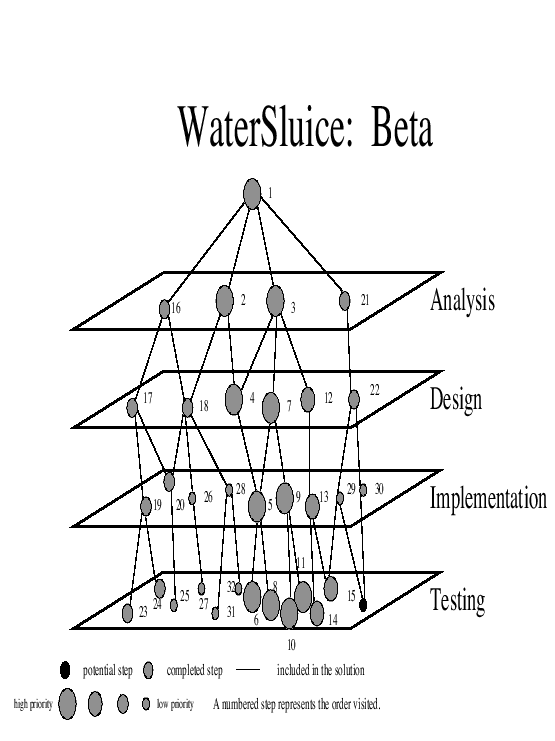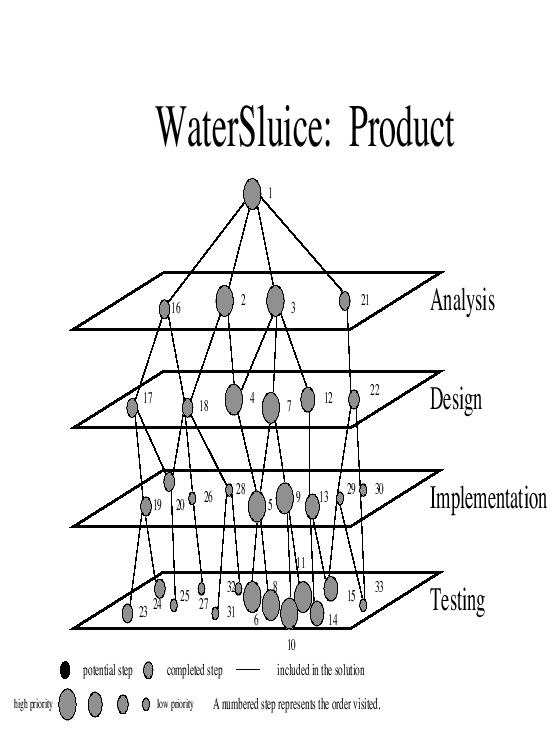Proof 4.1

The WaterSluice methodology leads to this sequence of steps.

step 1: Initial set up. PQ & = & a1

step 2: Remove a1 from the priority queue PQ. Expand all alternative steps near a1 using the function next and push them onto the priority queue PQ.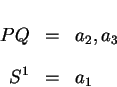At this stage there are two alternative next steps. Pick the next step with the highest priority. For clarity, assume this step is a2.
step 3: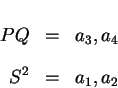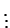step 11: The methodology is now at the proof of principle stage.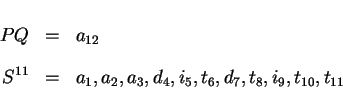step 15: The methodology is now at the prototype stage.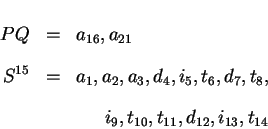step 24: The methodology is now at the alpha stage.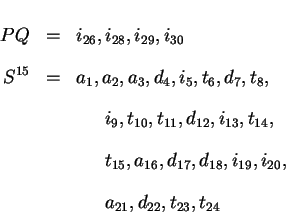step 32: The methodology is now at the beta stage.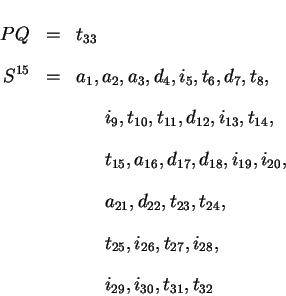step na + nd + ni + nt: The priority queue contains the last step of the final system acceptance test.
Thus, the WaterSluice software engineering methodology is static complete.

The WaterSluice software engineering methodology uses priority to guide the process combined with the iterative mechanism found in a cyclical methodology and governed by the steady progression found in a sequential methodology. If the environment is static, then all steps in the environment are known before the WaterSluice software engineering methodology begins. The WaterSluice software engineering methodology first discovers high priority steps from the analysis plane, the design plane, the implementation plane, and the testing plane. As higher priority steps are discovered and visited, they are eventually exhausted. This allows the lower priority steps to be visited. The WaterSluice software engineering methodology continues until the only remaining step to visit is the lowest possible priority step.

Corollary 4.1 (WaterSluice: Dynamic Complete)   The WaterSluice software engineering methodology is dynamic complete.

Proof 4.1.1   The WaterSluice software engineering methodology allows for the introduction of new information at every step and the removal of information that is no longer needed. Recall the presence of the priority queue. When steps are placed on the priority queue, the queue is rearranged. Low priority steps migrate to the end of the queue while high priority steps migrate to the beginning of the queue. Regardless of when a high priority item is discovered, the methodology will be able to react. For a dynamic environment, the WaterSluice software engineering methodology will eventually find the solution.

Corollary 4.2 (WaterSluice: Non-monotonic Complete)   The WaterSluice software engineering methodology is non-monotonic complete.

Proof 4.2.1   The WaterSluice software engineering methodology allows for the introduction of new information at every step and the removal of information that is no longer needed. Define a priority function that takes into account non-monotonic steps. Consistent steps are assigned similar high priority. Non-consistent steps are assigned lower priority. When steps are placed on the priority queue, the queue is rearranged. Low priority steps migrate to the end of the queue while high priority steps migrate to the beginning of the queue. Regardless of when a high priority item is discovered, the methodology will be able to react. For a non-monotonic space, the WaterSluice software engineering methodology will eventually find the solution leaving behind the conflicting steps.

Corollary 4.3 (WaterSluice: Best Case)   The best case performance of the WaterSluice software engineering methodology is O(1).

Proof 4.3.1   Let the environment consist of na analysis steps, nd design steps, ni implementation steps, and nt testing steps. Let the correct analysis step be the very first step a1. Let the correct design step be the very first step d1. Let the correct implementation step be the very first step i1. Let the system acceptance test be the very first step t1. To satisfy the problem statement would require one analysis, one design, one implementation, and one testing step. In this environment the WaterSluice software engineering methodology will require only four steps. Thus the performance is O(1).

Corollary 4.4 (WaterSluice: Worst Case)   The worst case performance of the WaterSluice software engineering methodology is O(N) where N is the size of the environment.

Proof 4.4.1   Let the environment consist of na analysis steps, nd design steps, ni implementation steps, and nt testing steps. Let the system acceptance test be the lowest priority step tn<<2119>>t. The number of steps to find a solution is na + nd + ni + nt which is O(N).

Corollary 4.5 (WaterSluice: Average Case)   The average case performance of the WaterSluice software engineering methodology is an order-of-magnitude less than N where N is the size of the environment.

Proof 4.5.1

Let d be the depth of the space. In the special case of this space d is 4. Let b be the average fan out of a step in the space if there was no priority function. If the space has N steps then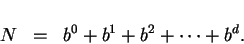To a reasonable approximation, only the last term is significant and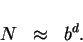Consider the addition of a priority function. The best case priority function would guide the algorithm directly to a solution. In this case, the fan out would be 1 and the total number of steps would be O(1). A worst case priority function would not guide the algorithm. Thus the fan out would be b and the total number of steps would be O(N). In the average case, the priority function would trim the fan out by half. Thus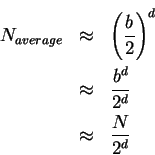On average the performance of the algorithm is an order-of-magnitude less than N.

Table 5.1: Summary of Completeness
 Methodology Static Complete Dynamic Complete Non-monotonic Complete sequential yes no no cyclical yes yes no WaterSluice yes yes yes

Table 5.2: Summary of Performance
 Methodology Best Worst Average sequential O(N) O(N) O(N) cyclical O(1) O(N) O(N) WaterSluice O(1) O(N) order-of-magnitude less than NNext: Summary Results from the Up: Supporting Theorems Previous: An Example of Cyclical
Ronald LeRoi Burback
1998-12-14